Aidan075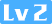186 0 0# 读取数据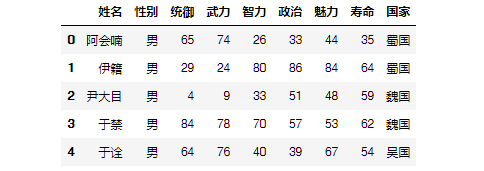#描述性分析
df.describe()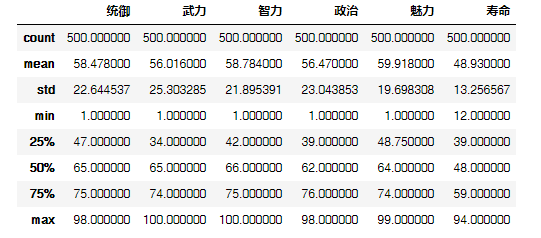• 平均数
1. 简单算数平均数，这里没什么好说的

2. 加权平均数，应用最广泛。这里举个栗子：武力值高不代表带领军队时的战力，不然关羽岂不是无敌，所以这时候用统御能力加权平均更合适。

3. 几何平均数，多用于流程转化中的平均，比如多步骤的转化率求平均值



data = df$'武力'$
#简单算数平均数
np.average(data)
#加权平均数
np.average(data,weights=df$'统御'$)
#几何平均数
pow(np.prod(data),1/len(data))


• 众数



counts = np.bincount(data)
np.argmax(counts)


• 分位数



# 中位数
np.median(data)
# 四分位数
np.percentile(data, (25, 50, 75), interpolation='midpoint')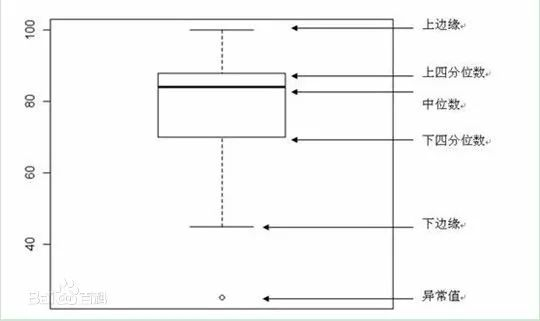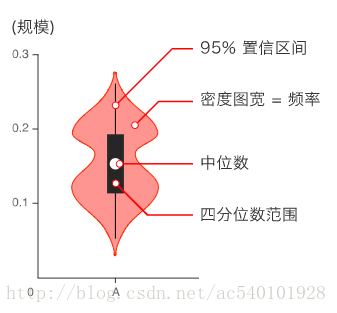• 极差



# 极差
np.max(df$'武力'$)-np.min(df$'武力'$)


• 方差、标准差



# 方差
np.var(df$'武力'$)
# 标准差
np.std(df$'武力'$)


• 平均差

• 异众比率

• 偏态系数

• 峰态系数



#偏度、峰度
from scipy import stats
x = df\_wu$'武力'$
skew = stats.skew(x)
kurtosis = stats.kurtosis(x)





df\_wei = df.loc$(df\['国家'$ == '魏国')\]
df\_shu = df.loc$(df\['国家'$ == '蜀国')\]
df\_wu  = df.loc$(df\['国家'$ == '吴国')\]
data = df\_wu$'武力'$
plt.hist(data,20,normed=True,facecolor='g',alpha=0.9)
plt.show()1. 从平均值看，蜀国武将的平均武力在三个国家之上

2. 从标准差看，吴>蜀>魏，这说明吴国人物间武力差距更大一些，而魏国人物武力分布较为均匀。

3. 从偏度上看：三国偏态系数均小于0，平均数在众数之左，是一种左偏的分布，又称为负偏。 从上面三个图中也可以看出：其中蜀国的武力分布众数偏在右侧更明显一点，长尾拖在左边。 从峰度上看：三国偏态系数均小于0，均是低峰态，相对来说蜀国人物武力分布较另外两国人物武将武力分布更窄一些。

PS:大家可能注意到求出的偏态系数为负数，这是因为在实际应用中，通常将峰度值做减3处理。

点击左下角原文链接，直接进入知识星球（免费）原贴获取文中涉及的三国数据和ipynb格式的python代码。

或者微信后台回复“统计分析”，也可同样获取。

《数据茶水间》-木东居士

《从零进阶！数据分析的统计基础》

《深入浅出统计学》